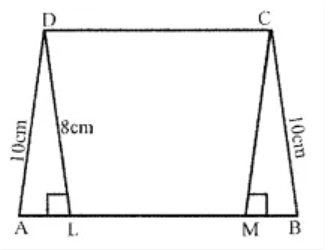# ML Aggarwal Solutions Class 8 Mathematics Solutions for Mensuration Exercise 18.1 in Chapter 18 - Mensuration

The perimeter of a trapezium is 52 cm. If its non-parallel sides are 10 cm each and its altitude is 8 cm, find the area of the trapezium.Given,

Perimeter of a trapezium = 52 cm

Length of each non-parallel side = 10 cm

Altitude DL = 8 cm

Now,

In right ∆DAL, by Pythagoras Theorem we have

DA2 = DL2 + AL2

(10)2 = (8)2 + AL2

100 = 64 + AL2

AL2 = 100 – 64 = 36 = (6)2

∴ AL = 6 cm

Similarly,

BM = 6 cm and DC = LM

Also, we have

Perimeter = AB + BC + CD + DA

and CD = DA

So, CD + DA = 2DA

But,

AB + CD = Perimeter – 2 AD

= 52 – 2 × 10

= 52 – 20

= 32 cm

Thus, the area of trapezium = ½ × (sum of parallel sides) × altitude

= ½ × 32 × 8

= 128 cm2

Related Questions

Lido

Courses

Teachers

Book a Demo with us

Syllabus

Maths
CBSE
Maths
ICSE
Science
CBSE

Science
ICSE
English
CBSE
English
ICSE
Coding

Terms & Policies

Selina Question Bank

Maths
Physics
Biology

Allied Question Bank

Chemistry
Connect with us on social media!